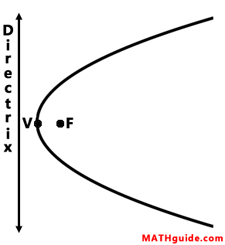Equations of Parabolas
View the Lesson | MATHguide homepage Updated June 18th, 2023

 Given: A parabola with the focus F(0, 7) and directrix x = -4.Calculate: The location of the vertex and the equation of the parabola.V(, )(y - + )2 = (x - + )